## A wheel of diameter 118 cm has an axle of diameter 5.8 cm. A force 160 N is exerted along the rim of the wheel. What force should be applied

Question

A wheel of diameter 118 cm has an axle of diameter 5.8 cm. A force 160 N is exerted along the rim of the wheel. What force should be applied to the outside of the axle in order to prevent the wheel from rotating? Assume the direction of this force to be tangential to the axle. Answer in units of N.

in progress 0
3 weeks 2021-08-25T02:33:23+00:00 1 Answers 0 views 0

The applied force should be of 3255N, in the opposite direction of the first one.

Explanation:

For the wheel to remain in rotational equilibrium, the sum of the torques acting on it must be equal to zero. Let F₁ be the force acting along the rim of the wheel, and F₂ the force applied to the outside of the axle, and r₁ and r₂ the distances from the center of the wheel and the rim and the axle, respectively. (Note that r₁ is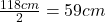and r₂ is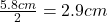because they are the radii, not the diameters). So, we can write the relationship: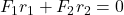Solving for F₂, we get: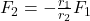Plugging in the given values, we have: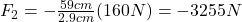This means that the force applied to the outside of the axle to prevent the wheel from rotating must be of 3255N. The negative sign means that the torque from this force has to be in the opposite direction of the first one, i.e, if F₁ acts in clockwise direction, F₂ must act in counterclockwise direction.